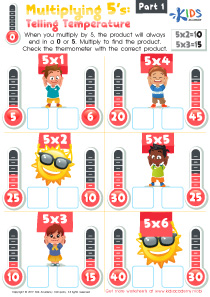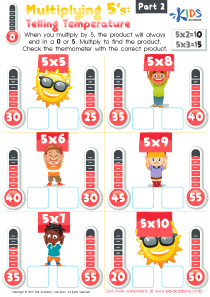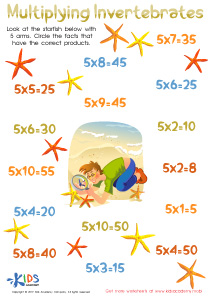# Math Lesson - Multiplication Facts 5, Grade 3

• ### Activity 1 / Multiplying 5’s: Telling Temperature WorksheetMultiplication problems are quite easily solved if you know some of the simple tricks and tips. For example, when multiplying 10’s the product always ends in a 0. That is just one trick you can teach your child. Another one, which they will learn in this worksheet, is that when you multiply by 5, the product will always end in a 0 or 5. Look at the problems in this printout. Help them multiply to find the product. Then, check the thermometer with the correct product.

• ### Activity 2 / Multiplying 5’s: Telling Temperature Part 2 WorksheetMultiplication problems are quite easily solved if you know some of the simple tricks and tips. For example, when multiplying 10’s the product always ends in a 0. That is just one trick you can teach your child. Another one, which they will learn in this worksheet, is that when you multiply by 5, the product will always end in a 0 or 5. Look at the problems in this printout. Help them multiply to find the product. Then, check the thermometer with the correct product.

• ### Activity 3 / Multiplying Invertebrates WorksheetBefore beginning this worksheet, take the time to explain to your kids what an invertebrate is. An invertebrate is an animal that does not have a backbone. Most animals and human beings have a backbone. Give your kids some common examples of an invertebrate such as the squid, jellyfish, and octopus. Now, look at the starfish in this picture with 5 arms. Help your kids circle the facts that have the correct products.

?